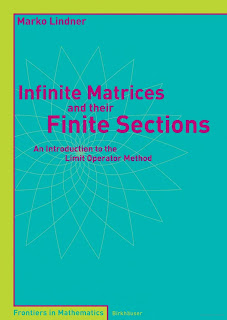MATRIX MATHEMATICS

# Infinite Matrices and their Finite Sections An Introduction to the Limit Operator Method Free DownloadThis book is concerned with the study of infinite matrices and their approximation by matrices of finite size. The main concepts presented are invertibility at infinity (closely related to Fredholmness), limit operators, and the stability and convergence of finite matrix approximations. Concrete examples are used to illustrate the results throughout, including discrete Schrödinger operators and integral and boundary integral operators arising in mathematical physics and engineering.